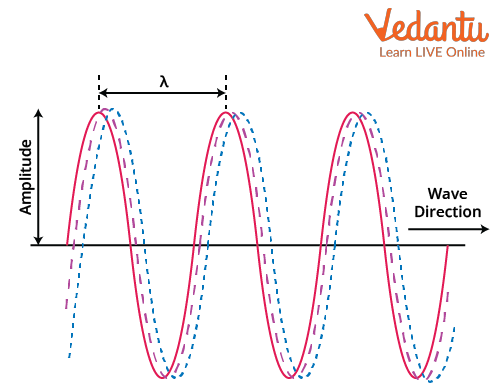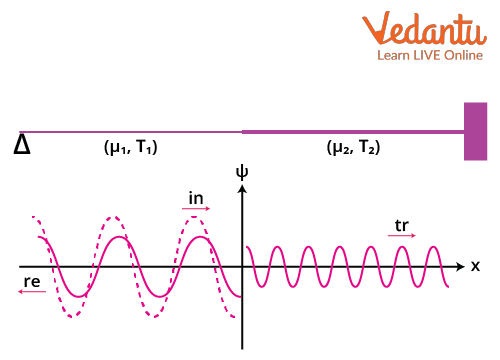Courses
Courses for Kids
Free study material
Free LIVE classes
More

# Reflection and Transmission of Waves on a String | JEELIVE
Join Vedantu’s FREE Mastercalss

## Introduction to Waves

What are waves? In simple words, the wave is just a disturbance. We all have come across waves in our lives, and we deal with them every day whether we realise it or not. Waves surround us every day. On a daily basis, we come into contact with many kinds of waves, including sine waves, stadium waves, seismic waves, cosine waves, and waves on a string. In addition to these waves, we also frequently experience other motions that are more accurately characterised as wavelike.  We could explain the motion of pendulums, the motion of any mass attached to a spring, the motion of swings, and some other periodic motions.

Waves can be defined as a disturbance that propagates energy and momentum from one place to another. This disturbance does not include the transport of the matter itself, but just the energy and momentum. Waves that require a medium for their transport are called mechanical waves and the ones that do not require a medium are known as non-mechanical waves.

## Sinusoidal Waves

Waves are of different types and in this article, we’ll be looking at the sinusoidal waves on a string. When we shake any string up and down, waves are formed in the shape of a sine function on the string. The oscillations in the string are the sine or cosine functions of time and the wave has a sinusoidal shape as we have already mentioned before. Since the wave moves forward, it is known as a travelling wave and the diagram of a travelling wave is shown in the diagram below.Travelling Sinusoidal Wave

## Mathematical Description of a Sinusoidal Wave

In the diagram, we can see the wavelength of the wave represented by $\lambda$ and it is the period of the wave in space. The point of minimum vertical amplitude is known as the trough and the point of maximum vertical amplitude is known as the crest. The wavelength is just the distance between two consecutive crests or troughs.

The absolute vertical displacement is known as the amplitude of the wave and is denoted by A. The time period is the time taken to travel a distance equal to the wavelength and is represented by T. Since the wave is moving forward, it has some velocity, and we represent the velocity by v. Frequency is the reciprocal of the time period. We can now write the relations between these variables of a wave.

\begin{align} &v=\dfrac{\lambda}{T} \\ \\ & \Rightarrow v=\lambda f \end{align}

The above equation is known as the fundamental equation of waves.

A travelling wave going towards the right can be represented by the equation,

$y=A \cos (k x-\omega t)$

Similarly, a wave travelling towards the left side will be represented by,

$y=A \cos (k x+\omega t)$

This is the transmission wave equation. Here y is the displacement of the particle of the string at a particular coordinate x and at time t. The variable k in the equation is called the wave number and ω is the angular frequency of the wave. We have the relations,

\begin{align} &k=\dfrac{2 \pi}{\lambda} \\ \\ &\Rightarrow \omega=2 \pi f \\ \\ & \Rightarrow v=\dfrac{\omega}{k} \end{align}

## Reflection and Transmission of Waves on a String

All the above description was of a wave travelling in open space in a single medium. When a wave contacts another medium, some waves will be reflected into the first medium and some will be transmitted into the second since wave speed relies on the characteristics of the media. We will study reflection and transmission now.Incident, Reflected, and Transmitted waves on a string

## Analysis of the Setup

We have taken two different strings having mass densities μ1 and μ2 respectively and have considered the tension to be the same in both strings for the sake of simplicity. The strings are tied by a knot. We send a wave towards the right by vibrator at the string shown by a triangle and this wave is the incident wave. The wave arrives at the knot and the knot itself vibrates due to the incoming incident wave. The knot then acts as a wave transmitter itself, and it sends a wave in both directions.

Wave reflection and transmission take place at the knot. The wave that it sends back to the left side is the reflected wave and the wave that it sends to the right side is the transmitted wave. In order to analyse the situation, we'll assume that the strings are very long and that we're looking at moments before the reflected wave reaches the vibrator. The transmitted wave is also presumed not to have hit the other end.

Let's say the vibrator is moving at a specific (angular) frequency. The frequencies of the incident, reflected, and transmitted waves will all be the same at that point. The wavenumbers and wavelengths of the waves moving in the same medium will be equal. In this case, the reflected and incident waves will have the same wavenumber and wavelength and the transmitted wave will have different wavenumbers and wavelengths. The reflection of waves inverts it.

The velocity in both the mediums can be given by,

\begin{align} &v_{1}=\sqrt{\dfrac{T}{\mu_{1}}} \\ \\ &v_{2}=\sqrt{\dfrac{T}{\mu_{2}}} \end{align}

## Boundary Conditions

Suppose we represent the sum of the incident and reflected wave by y1 and the transmitted wave by y2 then the boundary conditions state that the displacement of the string at the boundary at x = 0 must be the same. Here, we use y1 or y2 for the displacement at that location since the strings are continuous at the junction. Additionally, the force acting on the knot needs to be balanced.

This gives,

\begin{align} &y_{1}(0, t)=y_{2}(0, t) \\ \\ &\left(\dfrac{\partial y_{1}(0, t)}{\partial x}\right)_{x=0}=\left(\dfrac{\partial y_{2}(0,t)}{\partial x}\right)_{x=0} \end{align}

## Reflection and Transmission Coefficients

Let the incident, reflected, and transmitted waves be represented by yin , yr , and yt respectively. The equations for these waves will be,

\begin{align} &y_{i n}=A_{i n} \cos \left(k_{1} x-\omega t\right) \\ \\ &y_{r}=A_{r} \cos \left(k_{1} x+\omega t\right) \\ \\ &y_{t}=A_{t} \cos \left(k_{2} x-\omega t\right) \end{align}

K1 and k2 are the wave numbers of the different media.

Now we have,

\begin{align} &y_{1}=y_{i n}+y_{r} \\ \\ &y_{1}=A_{i n} \cos \left(k_{1} x-\omega t\right)+A_{r} \cos \left(k_{1} x+\omega t\right) \\ \\ &y_{2}=A_{t} \cos \left(k_{2} x-\omega t\right) \end{align}

We can simplify,

\begin{align} &y_{1}=A_{i n}\left(\cos k_{1} x \cos \omega t+\sin k_{1} x \sin \omega t\right)+A_{r}\left(\cos k_{1} x \cos \omega t-\sin k_{1} x \sin \omega t\right) \\ \\ &y_{1}=\cos _{1} x \cos \omega t\left(A_{i n}+A_{r}\right)+\sin k_{1} x \sin \omega t\left(A_{i n}-A_{r}\right) \\ \\ &y_{2}=A_{t}\left(\cos k_{2} x \cos \omega t+\sin k_{2} x \sin \omega t\right) \end{align}

Applying the first boundary condition we get,

\begin{align}&\left(A_{i n}+A_{r}\right) \cos \left(k_{1} \cdot 0\right) \cos \omega t+\left(A_{i n}-A_{r}\right) \sin \left(k_{1} \cdot 0\right) \sin \omega t=A_{t}\left(\cos \left(k_{1} \cdot 0\right) \cos \omega t+\sin \left(k_{1} \cdot 0\right) \sin \omega t\right) \\ \\&\left(A_{i n}+A_{r}\right) \cos \omega t=A_{t} \cos \omega t \\ \\ &A_{i n}+A_{r}=A_{t} \end{align}

Applying the second boundary condition gives,

\begin{align} &\left(\dfrac{\partial y_{1}}{\partial x}\right)_{x=0}=\left(\dfrac{\partial y_{2}}{\partial x}\right)_{x=0}\end{align}

\begin{align}&\left(A_{i n}+A_{r}\right)\left(-k_{1} \sin \left(k_{1} \cdot 0\right) \cos \omega t\right)+\left(A_{i n}-A_{r}\right)\left(k_{1} \cos \left(k_{1} \cdot 0\right) \sin \omega t\right)=A_{t}\left(-k_{2} \sin \left(k_{2} \cdot 0\right) \cos \omega t+k_{2} \cos \left(k_{2} \cdot 0\right) \sin \omega t\right) \\ \\ &\left(A_{i n}-A_{r}\right)\left(k_{1} \sin \omega t\right)=A_{t}\left(k_{2} \sin \omega t\right) \\ \\ &\left(A_{i n}-A_{r}\right) k_{1}=A_{t} k_{2} \end{align}

So we have equations,

\begin{align} &A_{i n}+A_{r}=A_{t} \\ \\ &k_{1} A_{i n}-k_{1} A_{r}=k_{2} A_{t} \end{align}

We can then simplify these expressions as,

\begin{align} &1+\dfrac{A_{r}}{A_{i n}}=\dfrac{A_{i}}{A_{i n}} \\ \\ &1+R=T \end{align}

Here R is the reflection coefficient and T is the transmission coefficient.

What is the reflection coefficient? We’re about to calculate it.

For the relation from the second boundary condition, we can write similarly,

\begin{align} &k_{1} A_{i n}-k_{1} A_{r}=k_{2} A_{t} \\ \\ &k_{1}-k_{1} \dfrac{A_{r}}{A_{i n}}=k_{2} \dfrac{A_{t}}{A_{i n}} \\ \\ &k_{1}-k_{1} R=k_{2} T .....(1) \end{align}

Now putting, T=1+R in the above equation we get,

\begin{align} &k_{1}-k_{1} R=k_{2}+k_{2} R \\ \\ &\left(k_{1}+k_{2}\right) R=k_{1}-k_{2} \\ \\ &R=\dfrac{k_{1}-k_{2}}{k_{1}+k_{2}} \end{align}

This is the reflection coefficient formula. The transmission coefficient can similarly be found by solving the equations found from the boundary conditions. Putting,

R=T-1 in equation (1) we get,

\begin{align} &k_{1}-k_{1} T+k_{1}=k_{2} T \\ \\ &T=\dfrac{2 k_{1}}{k_{1}+k_{2}} \end{align}

This is the transmission coefficient formula.

## Conclusion

Waves can be defined as a disturbance that transfers energy and momentum from one location to another is what is known as a wave. Only the energy and momentum are involved in this disturbance; no actual substance is being transported. Waves on a string are mechanical waves.

When two strings of different mass densities are tied together by a knot and if vibrations are given to one of the strings, then at the knot of both waves the knot will act as a transmitter. It will send waves in both directions and the wave which is sent back to the direction of incidence is known as reflected wave. The wave that is sent to the other side of the knot is known as the transmitted wave. Since the medium of both sides of the knot are different, they have different velocities, but their frequencies are the same as given before.

Reflection coefficient is defined as the ratio of the amplitudes of the reflected and incident waves. Similar to this, the transmission coefficient is defined as the ratio of the amplitudes of the transmitted and incident waves.

Last updated date: 30th Sep 2023
Total views: 3.3k
Views today: 0.03k

## FAQs on Reflection and Transmission of Waves on a String | JEE

1. What do you mean by standing waves?

Sometimes it is possible to get a string, cord, chain, or cable to vibrate in such a way that you are making a wave, but the wave doesn't spread. It simply sways back and forth while stationary. This kind of wave is known as the standing wave. It can be defined as a wave that oscillates in time, but the peak amplitude does not move in space. The peak amplitude of a standing wave at any point in space is constant with respect to time.

2. What is the weightage of Wave and Oscillations in JEE Main?

Wave and oscillation is an important topic, not just from the exam point of view but the applications that it has in various fields of Physics. Concepts studied in wave and oscillations are used in topics ranging from optics, electronics, RLC circuits, etc. It also has a weightage of around 3.3% in JEE main and one question is asked every year about this topic. This topic includes SHM, Beats, Doppler’s effect, etc.